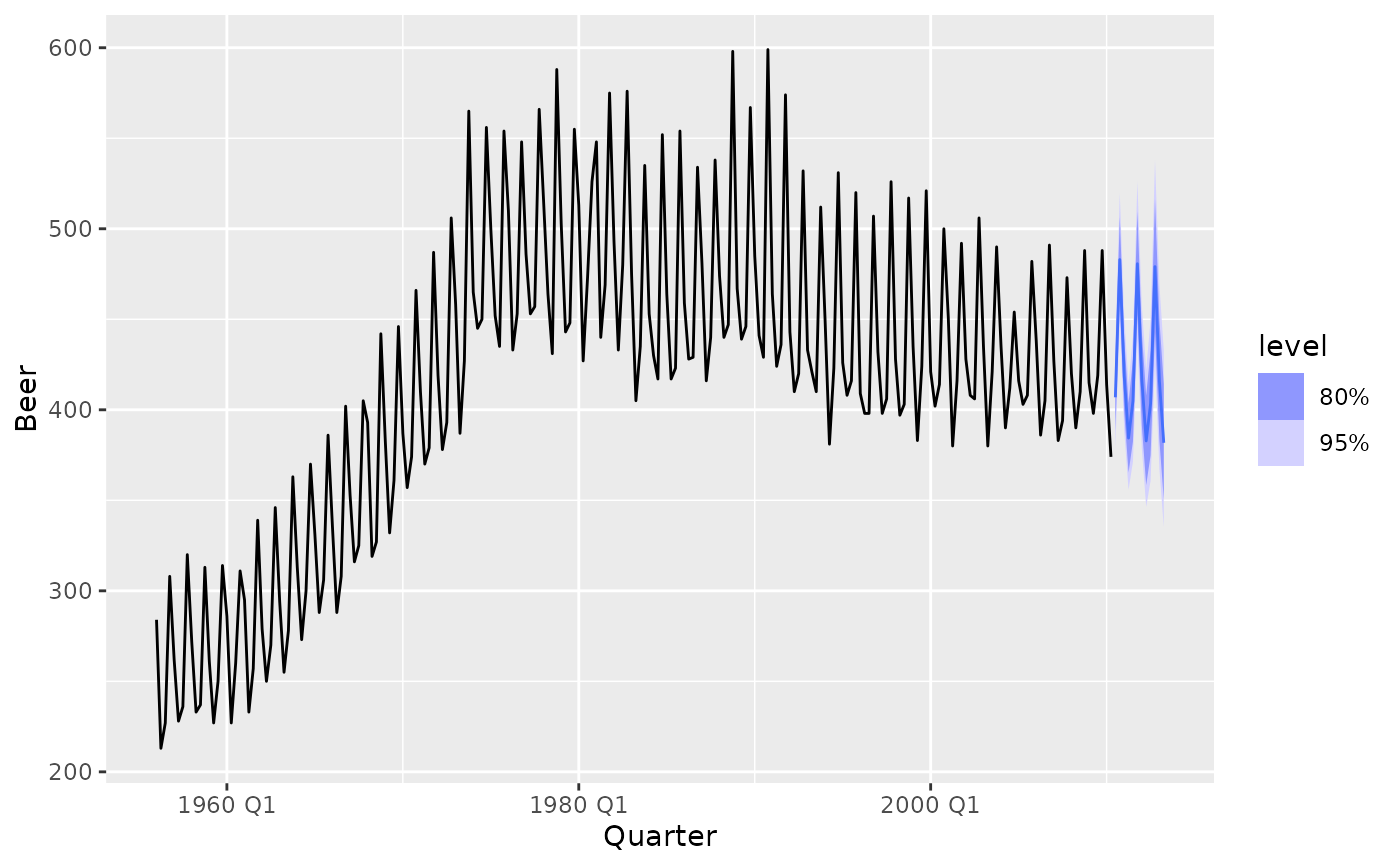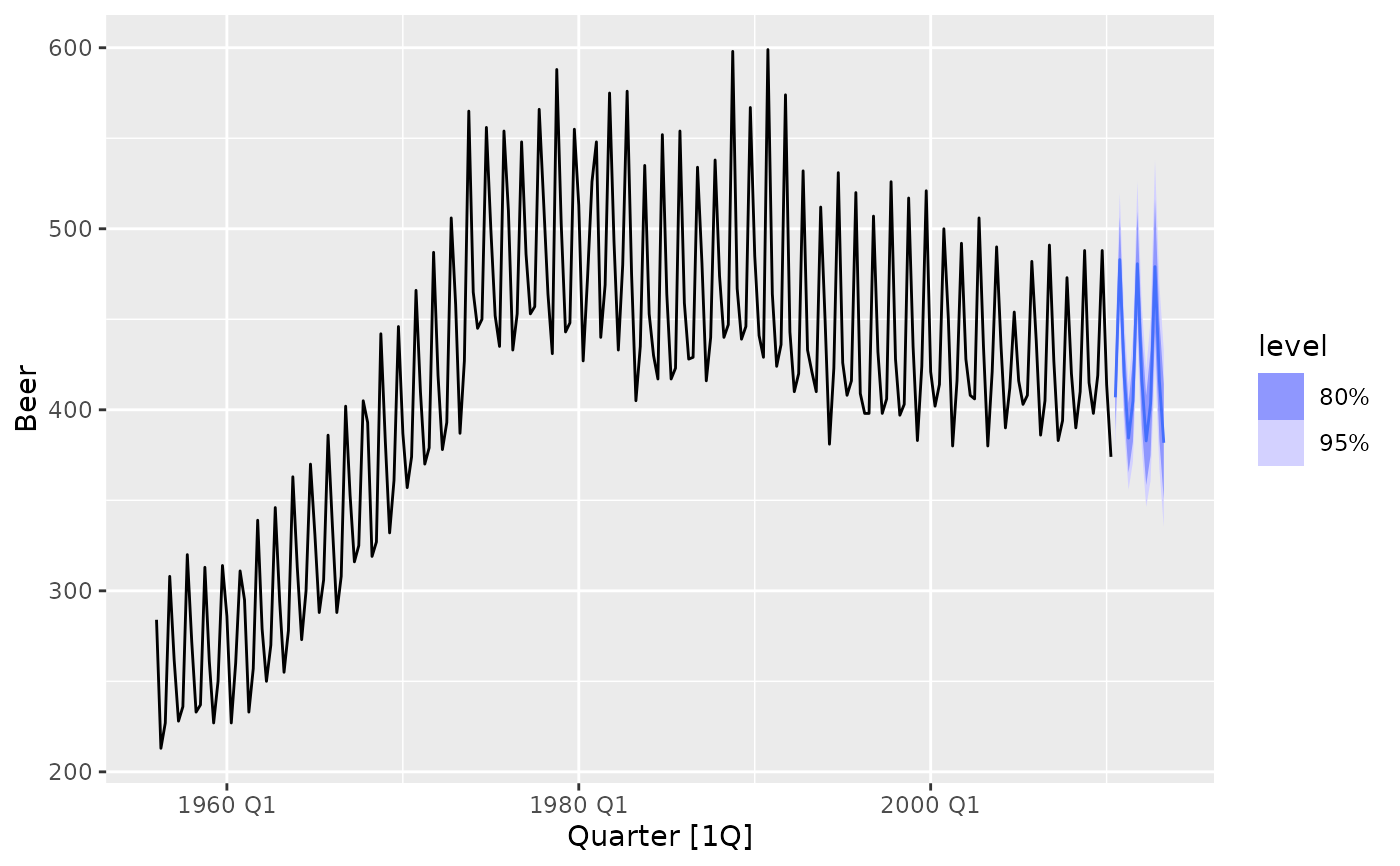Produces a forecast plot from a fable. As the original data is not included in the fable object, it will need to be specified via the data argument. The data argument can be used to specify a shorter period of data, which is useful to focus on the more recent observations.

# S3 method for fbl_ts
autoplot(object, data = NULL, level = c(80, 95), show_gap = TRUE, ...)

# S3 method for fbl_ts
autolayer(
object,
data = NULL,
level = c(80, 95),
point_forecast = list(mean = mean),
show_gap = TRUE,
...
)

## Arguments

object A fable. A tsibble with the same key structure as the fable. The confidence level(s) for the plotted intervals. Setting this to FALSE will connect the most recent value in data with the forecasts. Further arguments passed used to specify fixed aesthetics for the forecasts such as colour = "red" or size = 3. The point forecast measure to be displayed in the plot.

## Examples

library(fable)
library(tsibbledata)

fc <- aus_production %>%
model(ets = ETS(log(Beer) ~ error("M") + trend("Ad") + season("A"))) %>%
forecast(h = "3 years")

fc %>%
autoplot(aus_production)aus_production %>%
autoplot(Beer) +
autolayer(fc)